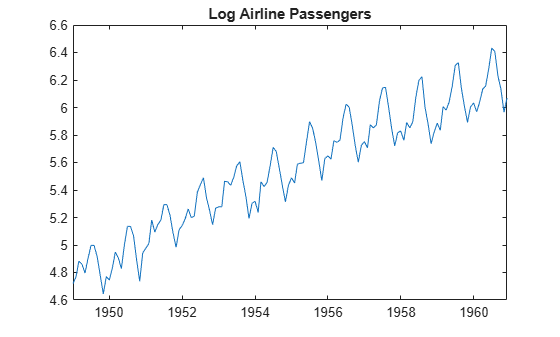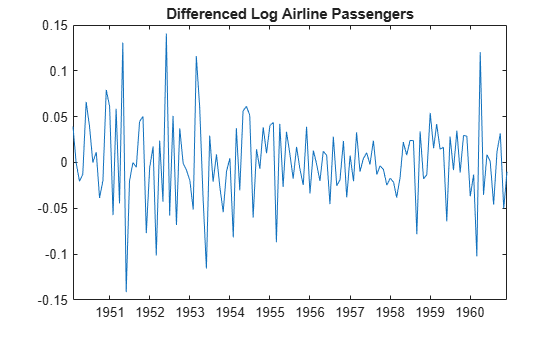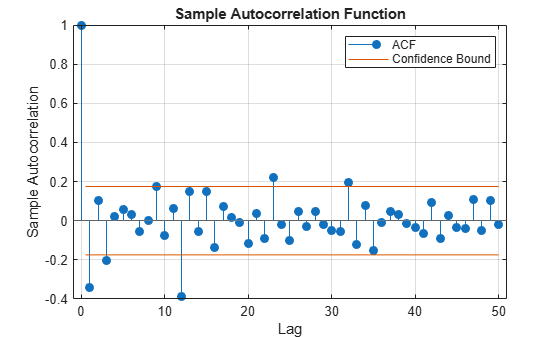Specify Multiplicative ARIMA Model

This example shows how to specify a seasonal ARIMA model using arima. The time series is monthly international airline passenger numbers from 1949 to 1960.

Load the airline data set, and then plot the natural log of the monthly passenger totals.

y = log(Data);
T = length(y);

figure
plot(dates,y)
xlim([1,T])
datetick('x','mmmyy')
axis tight
title('Log Airline Passengers')
ylabel('(thousands)')The data look nonstationary, with a linear trend and seasonal periodicity.

Plot the seasonally integrated series.

Calculate the differenced series, $\left(1-L\right)\left(1-{L}^{12}\right){y}_{t}$, where ${y}_{t}$ is the original log-transformed data. Plot the differenced series.

A1 = LagOp({1,-1},'Lags',[0,1]);
A12 = LagOp({1,-1},'Lags',[0,12]);
dY = filter(A1*A12,y);

figure
plot(dY)
title('Differenced Log Airline Passengers')The differenced series appears stationary.

Plot the sample autocorrelation function (ACF).

figure
autocorr(dY,'NumLags',50)The sample ACF of the differenced series shows significant autocorrelation at lags that are multiples of 12. There is also potentially significant autocorrelation at smaller lags.

Specify a seasonal ARIMA model.

Box, Jenkins, and Reinsel suggest the multiplicative seasonal model,

$\left(1-L\right)\left(1-{L}^{12}\right){y}_{t}=\left(1-{\theta }_{1}L\right)\left(1-{\Theta }_{12}{L}^{12}\right){\epsilon }_{t},$

for this data set (Box et al., 1994).

Specify this model.

Mdl = arima('Constant',0,'D',1,'Seasonality',12,...
'MALags',1,'SMALags',12)
Mdl =
arima with properties:

Description: "ARIMA(0,1,1) Model Seasonally Integrated with Seasonal MA(12) (Gaussian Distribution)"
Distribution: Name = "Gaussian"
P: 13
D: 1
Q: 13
Constant: 0
AR: {}
SAR: {}
MA: {NaN} at lag 
SMA: {NaN} at lag 
Seasonality: 12
Beta: [1×0]
Variance: NaN

The property P is equal to 13, corresponding to the sum of the nonseasonal and seasonal differencing degrees (1 + 12). The property Q is also equal to 13, corresponding to the sum of the degrees of the nonseasonal and seasonal MA polynomials (1 + 12). Parameters that need to be estimated have value NaN.

References:

Box, G. E. P., G. M. Jenkins, and G. C. Reinsel. Time Series Analysis: Forecasting and Control. 3rd ed. Englewood Cliffs, NJ: Prentice Hall, 1994.